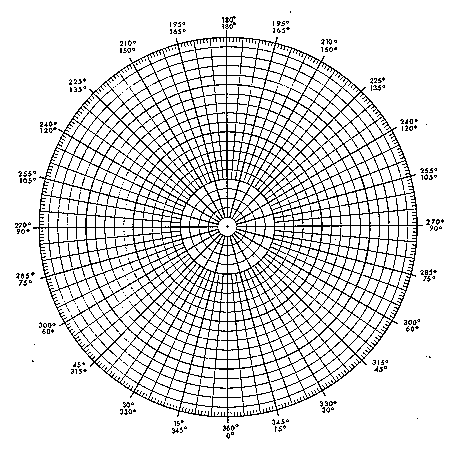The Loudness of Opera Singing

Material

One Sound Level Meter a.k.a. Decibel Meter (available at Radio Shack.)

a meter stick

Circular graph paper see below.

Part 1 Variations in Loudness

To Do and Notice

Set the decibel meter to the "A" scale and set it to "slow".

Stand 2 meters in front of the opera singer, let her or him sing a piece that she or he enjoys.

Notice the variation in loudness during the song.

Part 2 Sound level versus distance.

To Do and Notice

Set the decibel meter to the "A" scale and set it to "slow".

Mark distances at 4 meters, 2 meters and 1 meter from the singer. Mark 8 meters if you have enough space.

Stand 4 meters in front of an opera singer hold the decibel meter pointed toward the singer and have them sing a note.
Measure the loudness in decibels. Write the measurement in a table.

Quickly move from 4 meters to 2 meters distance from the singer.
measure the loudness in decibels.

Return to 4 meters and have them adjust the volume to the original measured decibel level if necessary, then quickly move to 1 meter and make another measurement.

Optional (repeat the measurement at 8 meters.)

Plot the graph of decibels versus distance

What's Going On?

The decibel meter is designed to model the perception of a human ear. The ear perceives the loudness of sound on a logarithmic scale stretching over twelve orders of magnitude from the quietest sound, known as the threshold of hearing to a sound so loud as to cause pain.

Theoretically the sound should expand in a hemisphere (ignoring walls.) Sound that expands in a sphere or a hemisphere follows the inverse square law.

Math Root

The intensity of sound is the power per meter squared, the loudness of sound is a human perception and is related to the intensity logarithmically. Each time 10 decibels are added to a loudness, the intensity of the sound increases by a multiple of 10. A scale is set by defining 0 decibels as the threshold of hearing, a loudness produced by 10^-12 watts/meter^2.

On this logarithmic decibel scale adding three decibels multiplies the intensity by two while subtracting three decibels cuts the intensity in half. Similarly adding 6 dB quadruples the intensity of a sound while subtracting 6 dB multiplies the loudness by one-fourth.

Thus when we double the distance between two loudness measurements the inverse square law would predict that the loudness should decrease to one fourth of its original loudness, which means that the decibel level will decrease by 6 dB.

The definition of the loudness, L, in decibels given the intensity I of sound is:

L = 10 log I/Io

where Io is 10^-12 W/m^2

and where this is the base 10 logarithm.

Etc.

OSHA standards for exposure to sounds recommend that people keep their exposure to sounds over long periods of time to less than 85 decibels.

Part 3, Variation in loudness with Distance

Measure the loudness of an opera singer as a function of angle. This is best done in a large room, such as an opera house, or outdoors.

Mark a circle around the opera singer at a radius of 4 meters. Define 0 degrees as directly in front of the singer.

As the opera singer holds a constant intensity note measure the loudness of the sound as a function of angle and then plot it on a polar graph , shown below. If you have two sound level meters you can use one to monitor the loudness of the note being sung by holding it in one place in front of the singer.

Notice the pattern of loudness versus angle. Notice how more sound is directed into the forward direction.

Repeat the exploration at the lowest frequency the singer can comfortably sing at, and then the highest frequency.

What's Going On?

The majority of the sound is emitted from the mouth.

For most frequencies of sound, the mouth is small compared to the wavelength of sound, and so the sound coming from the mouth diffracts as it exits the mouth spreading over a wide range of angles in the forward direction.

Math Root

An average human mouth is less than 8 cm in diameter, d = 8 cm.

The wavelength of sound, L, is L = v/f

where v is the velocity of sound, approximately 350 m/s

and f is the frequency.

a 100 Hz sound has a wavelength of L = 350/100 = 3.5 m

while a 1 kHz sound has a wavelength of 35 cm.

The wavelength of sound does not become comparable to the size of the human mouth until a frequency of 7 kHz is reached.

The angle between the forward direction and the first minimum in the diffraction pattern, T, is

sin(T) = L/d

For a 1 kHz sound, L/d is greater than 1 and there is no minimum.

For a sound with a wavelength equal to the diameter of the mouth, about 7 kHz, L/d = 1 and the angle to the first maximum is 90 degrees.

Etc.

The next time you are at an opera notice how the singers project their voices to the audience by turning to face the audience.Scientific Explorations with Paul Doherty © 2005 27 July 2005